Latest Banking jobs   »

# Quantitative Aptitude Quiz For Bank of Baroda AO 2023 -11th May

Q1. Mohit and Hemant can complete a work in 48 days, while Hemant and Vikash can do the same work in 36 days together. If efficiency of Vikash is 150% of Mohit then find time taken by Hemant to complete 3/4 th of the work?
(a) 144 days
(b) 108 days
(c) 96 days
(d) 36 days
(e) 120 days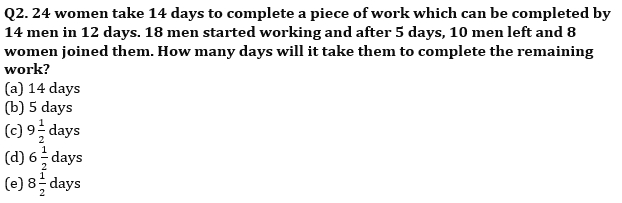Q3. An amount is invested at S.I. for three years at rate of 5% and S.I. received on that amount is Rs. 180. If the same amount is invested for two years at 10% C.I. for first year & R% C.I. for 2nd year & total C.I. received in two years is Rs. 318 then find value of R ?
(a) 15%
(b) 12%
(c) 22%
(d) 18%
(e) 25%

Q4. Cost price of an article is 39% less than the marked price of the article and shopkeeper earned 40% profit in selling the article. If amount of profit is Rs.196 more than amount of discount, then find cost price of article.
(a) Rs.1345
(b) Rs.1325
(c) Rs.1290
(d) Rs.1245
(e) Rs.1220

Q5. Hemant purchased some book and by selling 40% of total books he will get cost price of 80% books. If he sells 70% of remaining books at half of its initial profit percent and rest of the books remain unsold, find his overall profit %.
(a) 40%
(b) 45%
(c) 75%
(d) 43%
(e) 63%

Q6. An article is sold at 40% discount on M.P. and ratio of C.P. to S.P. is 5: 8 then what is the M.P. of article if the profit obtained on the article is Rs. 450.
(a) Rs. 1400
(b) Rs. 2400
(c) Rs. 1600
(d) Rs. 2200
(e) Rs. 2000

Q7. The ratio between perimeter of an equilateral triangle and a square is 3 : 8. The area of square is 800% of area of a rectangle having sides 8 m and 4 m. Find the perimeter of triangle.
(a) 22 m
(b) 20 m
(c) 24 m
(d) 28 m
(e) 32 m

Q8. The circumference of two circles is 176 cm and 220 cm respectively. There is a square whose side is 36 cm less than sum of the radius of both the circles. Then, find the area of that square (in cm²)?
(a) 841
(b) 784
(c) 676
(d) 729
(e) 625
Q9. Two pipes P and Q alone can fill a tank in 24 min and 30 min respectively and a pipe R alone can empty the tank by throwing out 8 gallons in 2 minutes. All the three pipes working together can fill the tank in 20 minutes. Find the capacity of the tank?
(a) 80 gallons
(b) 160 gallons
(c) 320 gallons
(d) 240 gallons
(e) None of these

Q10. Two cards are drawn at random from a pack of 52 cards, then find the probability of getting one red face card and one black ace?
(a) 1/221
(b) 2/221
(c) 76/221
(d) 9/221
(e) 5/221

Solutions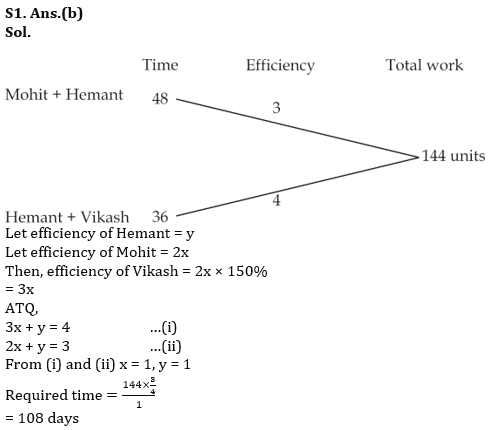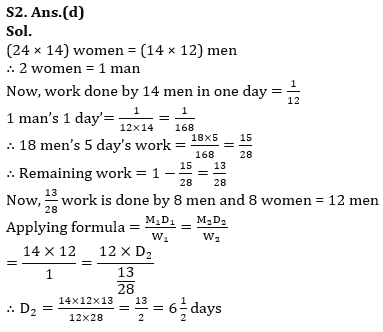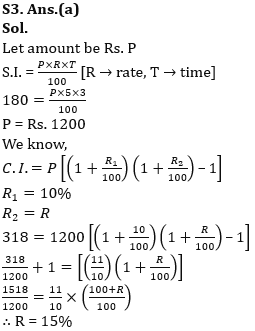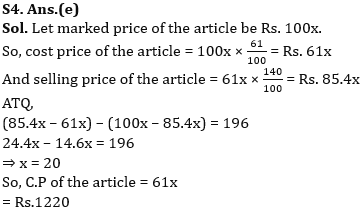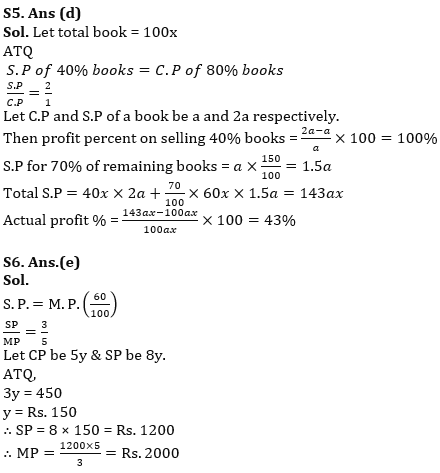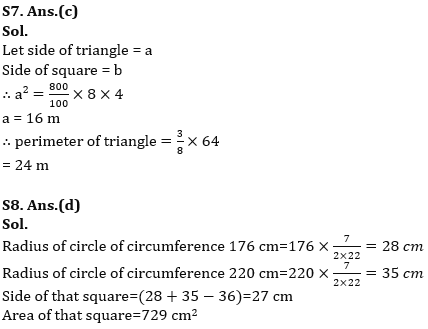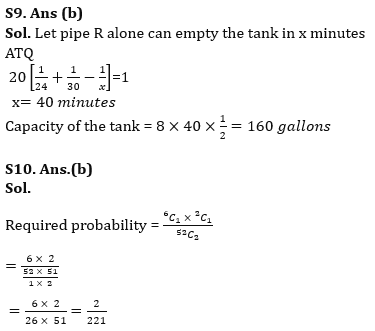.

## FAQs

### What is the selection process of Bank of Baroda AO 2023?

The selection process of Bank of Baroda AO consists of Online exam and Interview

#### Congratulations!Union Budget 2023-24: Free PDF